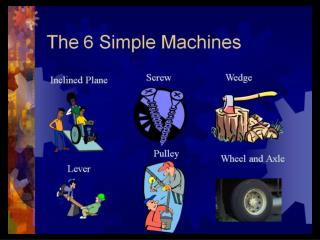DownloadDownload PresentationKey

# Key

Download Presentation## Key

- - - - - - - - - - - - - - - - - - - - - - - - - - - E N D - - - - - - - - - - - - - - - - - - - - - - - - - - -
##### Presentation Transcript

1. Key POINTS Simple machines Sowing machines, washer machine, coffee machine, etc. all involve the use of a machine to perform a variety of operations in domestic or industrial situations. The machines themselves are made of many parts and pieces. Simple machine is a term used to describe certain parts and pieces. A simple machine is an apparatus that is made of a small number of pieces and permits us to use more efficiently or more comfortably mechanical energy. It helps us reduce the necessary force to perform a task.

2. Key POINTS Simple machines • These machines may help us change the direction or the nature of an action, for instance, movement. • We will see in this section six types of simple machines that are frequently used: • Inclined plane • Wedge • Wheel and axle • Levers • Pulley

3. Key POINTS Simple machines Levers: a lever is solid bar that rotates, or turns around a fixed point. The bar can be straight or curved. The fixed point is called the fulcrum. A lever can multiply the strength of a force applied to an object. If you apply a force downward on one end of a lever, the other end can lift a load. There are three types of levers: a) First-class lever: A fulcrum is located between an input force and an output force. An example for this is a crow bar. INPUT FORCE OUTPUT FORCE FULCRUM

4. Key POINTS Simple machines b) Second-class lever: The output force is located between the input force and the fulcrum. We use this type of lever if we need a greater output force. An example of this type of lever is a bottle-top opener. OUTPUT FORCE INPUT FORCE

5. Key POINTS Simple machines c) Third-class lever: The input force is located between the output force and the fulcrum. Use this type of lever to reduce the distance over which you apply the input force or increase the speed of the end of the lever. OUTPUT FORCE INPUT FORCE

6. CATAPULTS: LEVERS

7. Some MATH F d 1 1 F d 2 2 Lever mathematics There is a mathematical relation that can be used to calculate the amount of force needed to lift an object: F1x d1=F2xd2 Where F is force measured in Newtons (N) and d is distance measured in meters (m) A force needed to lift an object is equal to the weight of that object in Newtons.

8. MOTOR FORCE F 2 F FORCE OF RESISTANCE 1

9. F F F F 1 2 1 2 FIRST SITUATION SECOND SITUATION A rock weighing 100 N (F1) is placed on the left side at one meter from the fulcrum. Calculate the Motor force (F2) knowing that there is a distance of two meters between the fulcrum and the motor force That same rock is now placed at two meters away from the fulcrum. Calculate the force of resistance (F1) knowing that there is a distance of two metersbetween the fulcrum and the force of resistance. F1x d1=F2xd2 100 N x 1 m = F2 x 2 m F2 = 50 N F1x d1=F2xd2 100 N x 2 m = F2 x 1 m F2 = 200 N

10. What is the distance between the fulcrum and the input force (75 N) knowing that the output force is 50 N and the distance is 7 meters? What input force is needed to lift a 60 N object, knowing that the distance from the fulcrum for the object is 2.5 meters and your distance from the fulcrum is 6 meters? Calculate the weight of your younger sister sitting on a see-saw knowing that you need 30 N to lift her. Your position is 3 meter from the fulcrum and she is sitting at 6 m away from the fulcrum. In addition, calculate the amount of your work on your behalf.

11. FIXED PULLEY MOVABLE PULLEY

12. Key POINTS Simple machines Inclined plane or ramp: a ramp is an inclined surface placed at an angle with the horizontal surface. It helps us in reducing the force needed to lift an object upward or to lower this object.

13. In the first situation, the force exerted to lift the heavy box is equal to the mass of that box. In the second and third situation, by using a ramp, you exert less force in lifting and displacing the box, but you need more distance to perform this task. It is also important to note that having a smaller angle between the ramp and the ground helps us in using less force to perform the task.

14. Key POINTS Simple machines Wedge: a wedge is a simple machine that has a thick end and a thin end. They are used to cut, split or pierce objects and to hold objects together. Inclined planes are stationary (Don’t move) whereas wedges can move to do work.

15. Key POINTS Simple machines Wheel and axle: a wheel and axle is a simple machine made of a wheel attached to a shaft, or axle. They act like a rotating collection of levers. The axle at the wheel’s center is like a fulcrum. Some examples are bicycle and car wheels, screwdrivers, steering wheels, doorknobs and electric fans.